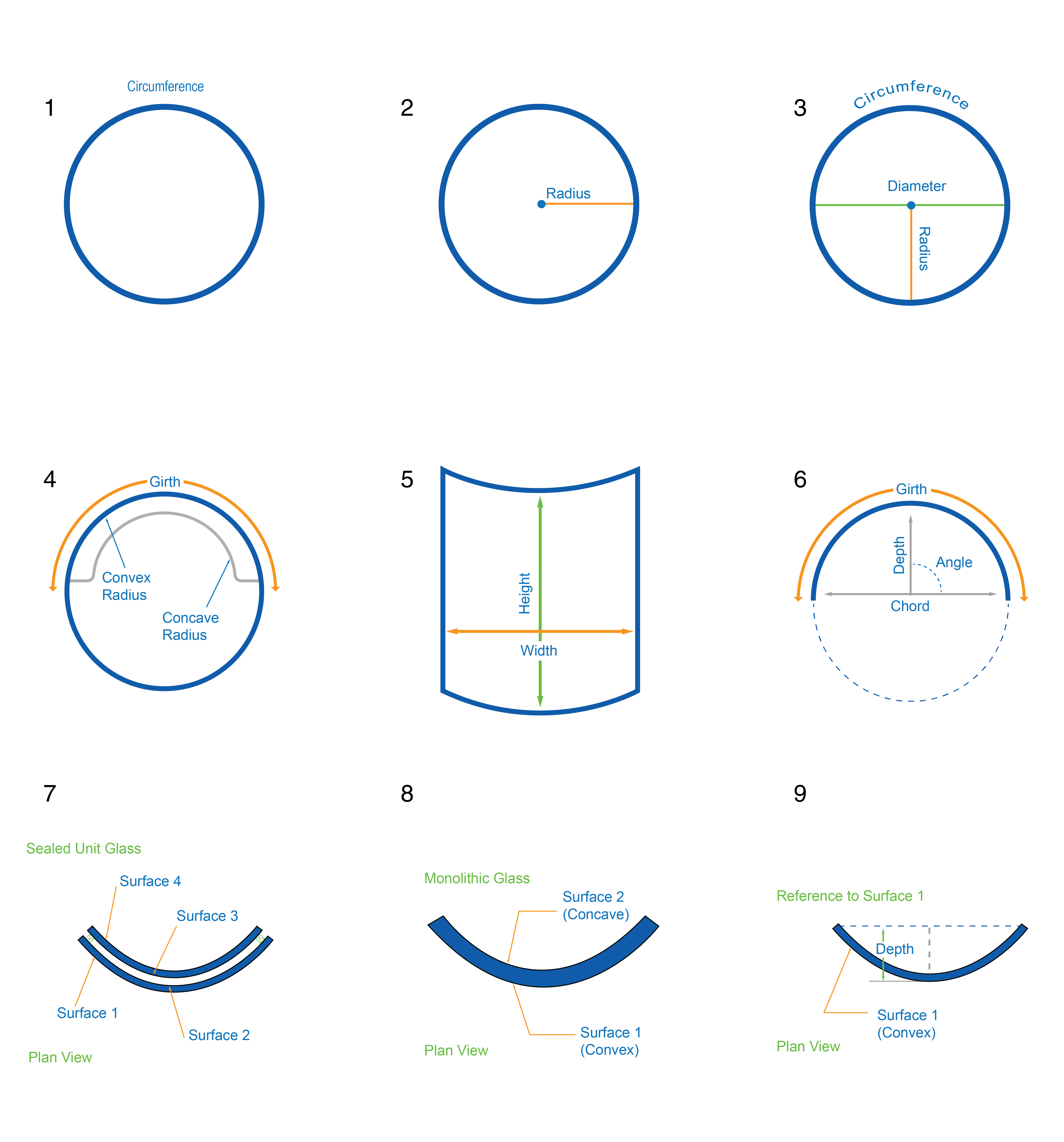# Definitions

## Important Definitions for Bent Glass1. Circumference: The distance around the outside of a circle.
2. Radius: A straight line from the center to the circumference of a circle. When specifying a radius, always specify which surface the radius is being measured to.
3. Diameter: The diameter is the length of the line through the center that touches two points on the edge of the circle.
4. Girth: Length around a curve. Measurement taken on either the concave or convex face of the glass (specify which). Measurement must be taken with a flexible (cloth) tape.
5. Chord: Line segment between two points on a given curve – measurement must be taken with a flat ruler.
6. Depth: Distance between the chord and the highest point of the curve. Maximum depth is 26″ (650mm).
7. Angle: Angle measurement in degrees of the bend.
8. Height: The length measurement of the edge perpendicular to the horizontal arc or girth. Maximum width is 118″ (3000mm). Maximum height is 110″ (2800mm).
9. Glass Surface: Insulated glass surfaces in relation to bent glass.